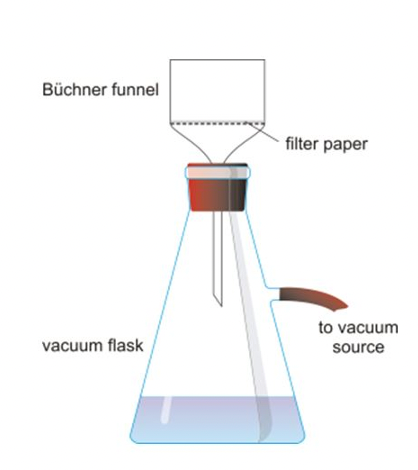# What is Gravimetric Analysis?

This is part of the HSC Chemistry course under Module 8 Section 1: Analysis of Inorganic Compounds. This section examines two commonly used quantitative techniques: gravimetric analysis and precipitation titration. Both techniques can be used to measure the concentration of inorganic substances.

### Gravimetric Analysis: Precipitation and Volatilisation

This video explores quantitative techniques for measuring ions, including two types of gravimetric analysis for the HSC: precipitation and volatilisation.

## What is Gravimetric Analysis?

• Gravimetric analysis is a quantitative technique and involves measuring the mass of a particular substance called the analyte. This usually involves first isolating the substance of desire from the chemical it is in.
• The type of gravimetric analysis which is utilised depends on the physical and chemical properties of the chemicals.

Precipitation Gravimetric Analysis

• Aims to quantify the analyte by measuring the mass of precipitate formed from it.
 Generally, precipitation gravimetric analysis follows these main steps: Measure the mass of original sample that contains the analyte Dissolve the sample in a suitable solvent and filtrate the solution Add a suitable chemical to the filtered sample to induce precipitation of the analyte Filtrate the new solution (using Büchner funnel/sintered crucible) to isolate the precipitate Dry the precipitate to remove residual water content Measure the mass of the precipitateExample 1 – Fertiliser analysis Fertilisers usually contain sulfate and phosphate ions   Assumptions: Only sulfate ions in the fertilisers are precipitated by barium ions All of the precipitate is collected by the filter paper• Calculation

A 0.4550 g solid mixture of fertiliser containing CaSO4 is dissolved in water and treated with an excess of Ba(NO3)2, resulting in the precipitation of 0.6168 g of BaSO4. What percentage by mass of the fertiliser was CaSO4?

Gravimetric analysis – BaSO4 is weighed to provide information on the amount of SO42– in the fertiliser.

Balanced equation of precipitation:

$$CaSO_4(aq) + Ba(NO_3)_2(aq) \rightarrow BaSO_4(s) + Ca(NO_3)_2(aq)$$

The molar ratio between BaSO4 and CaSO4 is 1:1, so the number of moles of BaSO4 = moles of CaSO4

$$n(BaSO_4) = \frac{0.6168}{137.3+32.07+4\times 16.00} = 0.002643\hspace{0.1cm}mol$$

$$n(CaSO_4) = 0.002643 \hspace{0.1cm}mol$$

$$mCaSO_4 = 0.002643 \times (40.03 + 32.07 + 4 \times 16.00) = 0.3598 \hspace{0.1cm} g$$

$$mass\% = \frac{0.3598}{0.4550} \times 100 = 79.08\% \hspace{0.1cm} (4s.f.)$$

• Example 2 – Laundry powder analysis

The phosphate content of a laundry powder was determined as follows. 6.85 g of the powder was dissolved in approximately 500 mL of water. A slight excess of a solution of magnesium chloride in an ammonia/ammonium chloride buffer was added, with stirring, until precipitation was complete. The precipitate was filtered, dried and its mass determined. The mass of precipitate was found to be 1.89 g.

The precipitate is magnesium ammonium phosphate hexahydrate whose formula is Mg(NH4)PO4.6H2O)

Calculate the number of moles of phosphorus in the precipitate and hence the percentage by

mass of phosphorus in the original laundry powder.

$$n(precipitate) = \frac{1.89}{24.31+14.01+4 \times 1.008+30.97+4 \times16.00 + 6(2\times1.008 + 16.00)}$$

$$n(precipitate) = 0.00771 \hspace{0.1cm} mol$$

Since in every mole of precipitate, there is one mole of phosphorus (atom), the number of moles of phosphorus = 0.00771 mol.

$$mass(phosphorus) = 0.00771 \times 30.97 = 0.239 \hspace{0.1cm}g$$

$$mass\% = \frac{0.239}{6.85} \times 100 = 3.49 \% (3s.f.)$$

Volatilisation Gravimetric Analysis

• Aims to quantify the analyte by measuring the change in mass of a particular substance when heat is applied.
• Mass loss due to gas leaving the system used for quantification
• Assumptions:
• substance e.g. acid is added in excess
• mass loss due to gas produced from reaction only

• Example 1 Analysis of NaHCO3 in baking soda
• reacting with excess acid and measuring mass loss due to CO2

The amount of sodium hydrogen carbonate in a 1.00 g sample of baking soda is analysed by reacting with excess sulfuric acid. The mass change before and after the reaction is 0.260 g.

Calculate the percentage by mass of sodium hydrogen carbonate in the baking soda.

$$2NaHCO_3(aq) + H_2SO_4(aq) \rightarrow 2CO_2(g) + 2H_2O(l) + Na_2SO_4(aq)$$

Mass of carbon dioxide produced

$$m(CO_2) = 0.260g$$

$$n(CO_2) = \frac{0.260}{12.01+2 \times 16.00} = 5.91 \times 10^{-3}$$

Since the stoichiometric ratio of sodium hydrogen carbonate to carbon dioxide is 1:1 the number of moles of sodium hydrogen carbonate is equal to the number of moles of carbon dioxide.

$$\therefore n(NaHCO_3) = 5.91 \times 10^{-3} mol$$

$$m(NaHCO_3) = 5.91 \times 10^{-3} \times (22.99+1.008+12.01+3 \times 16.00) = 0.496$$

$$%(\frac{m}{m}) = \frac{0.496}{1.000} \times 100 = 49.6%(3s.f)$$